Create a new printableMath Worksheets
Division

Sample - Click above to make a new math worksheet (PDF).
 Name _____________________________Date ___________________
Division
Complete.

1.
Divide 12 strawberries into groups of 3.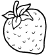____ groups _____ left over 12  ÷  3  =  ____
 2 * This is a pre-made sheet.Use the link at the top of the page for a printable page.
3.
Divide 13 raindrops into groups of 6.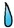____ groups _____ left over 12  ÷  6  =  ____
4.
Divide 19 snowflakes into groups of 9.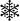____ groups _____ left over 18  ÷  9  =  ____
5.
Divide 14 ants into groups of 3.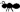____ groups _____ left over 12  ÷  3  =  ____
6.
Divide 31 baseballs into groups of 4.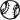____ groups _____ left over 28  ÷  4  =  ____